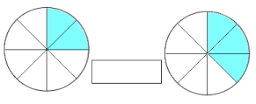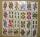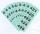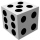# Probability

How probable is a randomly selected three-digit number divisible by five or seven?

Correct result:

p =  31.3333 %

#### Solution:We would be pleased if you find an error in the word problem, spelling mistakes, or inaccuracies and send it to us. Thank you!Tips to related online calculators
Would you like to compute count of combinations?

#### You need to know the following knowledge to solve this word math problem:

We encourage you to watch this tutorial video on this math problem:

## Next similar math problems:

• Air draftThe numbers 1,2,3,4,5 are written on five tickets on the table. Air draft randomly shuffled the tickets and composed a 5-digit number from them. What is the probability that he passed: and, the largest possible number b, the smallest possible number c, a
• Twenty-fiveHow many three-digit natural numbers are divisible by 25?
• Three digits numberFrom the numbers 1, 2, 3, 4, 5 create three-digit numbers that digits not repeat and number is divisible by 2. How many numbers are there?
• Three-digit integersHow many three-digit natural numbers exist that do not contain zero and are divisible by five?
• Inverted nineIn the hotel,, Inverted nine" each hotel room number is divisible by 6. How many rooms we can count with three-digit number registered by digits 1,8,7,4,9?
• RectangleIn rectangle with sides, 6 and 3 mark the diagonal. What is the probability that a randomly selected point within the rectangle is closer to the diagonal than to any side of the rectangle?
• Divisible by nineHow many three-digit natural numbers in total are divisible without a remainder by the number 9?
• Family94 boys are born per 100 girls. Determine the probability that there are two boys in a randomly selected family with three children.
• 7 digit numberIf 3c54d10 is divisible by 330, what is the sum of c and d?
• RecordsRecords indicate 90% error-free. If 8 records are randomly selected, what is the probability that at least 2 records have no errors?
• Divisible by 5How many three-digit odd numbers divisible by 5, which are in place ten's number 3?
• PIN codePIN on Michael credit card is a four-digit number. Michael told this to his friend: • It is a prime number - that is, a number greater than 1, which is only divisible by number one and by itself. • The first digit is larger than the second. • The second d
• CombinationsHow many different combinations of two-digit number divisible by 4 arises from the digits 3, 5 and 7?
• Three redsWhat is the probability that when choosing 3 carats from seven carats, all 3 reds will be red?
• How many 4How many 4 digit numbers that are divisible by 10 can be formed from the numbers 3, 5, 7, 8, 9, 0 such that no number repeats?
• RaffleIn the pool is numbers from 1 to 115. What is the probability that a randomly selected number is not a prime number?
• One diceCalculate the probability of a roll of one dice with the numbers 1, 2, 3, 4, 5, 6 on the walls. Write the results in a notebook in the shape of a fraction in the basic form: 2/3. a, The number 1 falls on the cube. b, The number 5 falls on the cube. c, An